GeeksforGeeks App
Open AppBrowser
Continue

# Row wise operation in R using Dplyr

The dplyr package in R programming is used to perform simulations in the data by performing manipulations and transformations. It can be installed into the working space using the following command :

`install.packages("dplyr")`

## Create Dataframe using Row

The data frame created by tibble contains rows and columns arranged in a tabular structure. It illustrates the data type of the data frame’s column. It can be created in R using the following dimensions

## R

 `# Using the required libraries``library``(``"dplyr"``)`` ` `# Declaring a tibble``data = ``tibble``(col1=``c``(1, 4, 2, 5, 6, 9, 5, 3, 6, 3),``              ``col2=``c``(``"a"``, ``"b"``, ``"a"``, ``"c"``, ``"b"``,``                     ``"b"``, ``"b"``, ``"a"``, ``"c"``, ``"a"``),``              ``col3=``c``(3, 2, 4, 2, 1, 4, 8, 6, 4, 2))`` ` `# Arranging data rowwise``data % > % ``rowwise``()`

Output: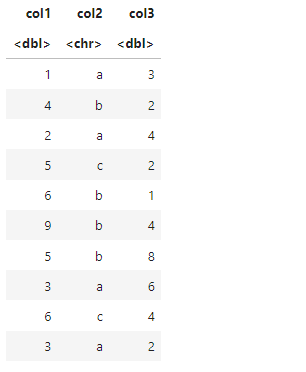## Application of the mutate method

The mutate() method in R is then applied using the pipe operator to create new columns in the provided data. The mutate() method is used to calculate the aggregated function provided.

Syntax: mutate(new-col-name = func)

Arguments :

• new-col-name – The new column to be added to the data
• func – The function to be applied on the specified data frame.

The following code snippet illustrates the procedure where the mean of col1 and col3 values of the data are calculated the same mean value is returned in all of these rows since group_by method is not taken into account.

## R

 `#computing the mean ``data %>%  ``mutate``(mean = ``mean``(``c``(col1,col3)))`

Output: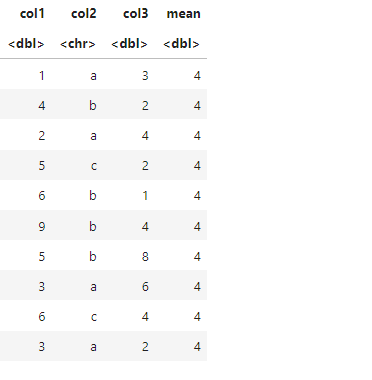## Using a combination of rowwise() and mutate() methods

In the following code snippet, the rowwise method is used in collaboration with the mutate method. Therefore the mean value of col1 and col3 value of the data table is calculated for each row individually. For instance, the mean of 1 and 3 in row 1 of the table is equivalent to 2 and is therefore displayed under the mean column.

## R

 `# Computing the mean ``data %>%  ``rowwise``() %>% ``mutate``(mean = ``mean``(``c``(col1,col3)))`

Output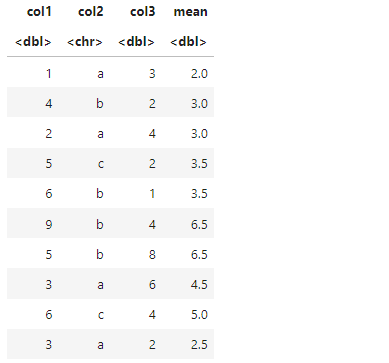## Using summarise method

The summarise method is used to create a summary of the values across the data rows that fall within one column. It is preferably used with a group_by method and the output data contains one row for each of the groups present in the column for which the group_by method is invoked. The method has the following syntax:

Syntax : Summarise(new-col-name=fun())

Arguments: fun – any aggregate function that may be applied over the rows

In the following code snippet, a new column sum is displayed which contains the submission of the values present in the col1 and col3 values of the data. The sum aggregate method has been used to calculate the total values.

## R

 `# Computing the mean ``data %>% ``  ``rowwise``() %>% ``  ``summarise``(sum = ``sum``(``c``(col1,col3)))`

Output: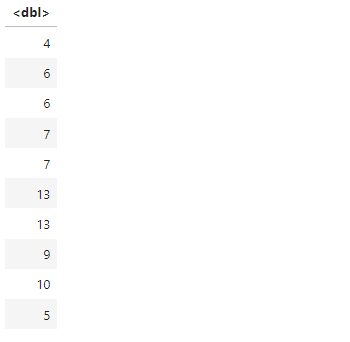## Using summarise in combination with group_by

To apply a function to every group in the data, we need to first group the data according to the classes available. The group_by() method in the dplyr package divides the data into different segments. It has the following syntax :

Syntax: group_by(col1, col2..)

Arguments : col1, col2,.. – The columns to group the data by

In the following code snippet, the group_by method is combined with a summarise method to calculate the sum of the grouped col3 values

For Example, the value 4 appears 3 times in the col3 parameter and has been returned in the output only once.

## R

 `# Computing the summary``data %>% ``  ``rowwise``() %>% ``  ``group_by``(col3) %>% ``  ``summarise``(sum = ``sum``(``c``(col1,col3)))`

Output: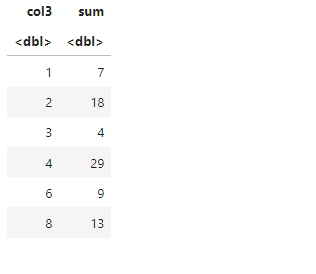## Using across method

The Across method is used to span multiple data elements be its rows, or columns of the data. For instance, it can be used to check as well as return the desired output with various inbuilt functions like is.numeric. In the following code, the row sums of all the rows have been calculated which contain integral values satisfying the condition of being numerical. Therefore, the sum of col1, col3, and col4 values for each row has been displayed.

## R

 `# Applying across``data %>% ``mutate``(sum = ``rowSums``(``across``(``where``(is.numeric))))`

Output: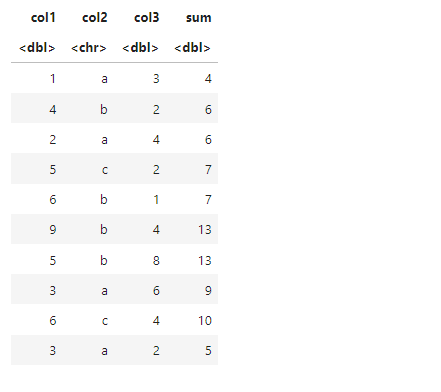The do method is used to perform a specific task of returning a subset of values of the data frame by applying methods like head over it. The head(.,1) is used to print the first row of every group contained in the group_by method.

## R

 `# Head method``data %>% ``group_by``(col3) %>% ``do``(``head``(., 1))`

Output: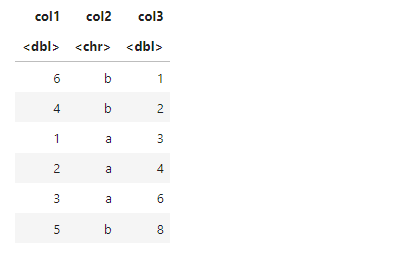My Personal Notes arrow_drop_up